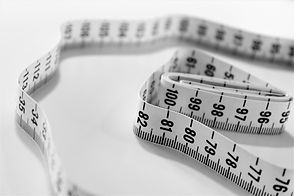# How to measure for your End 2 End Swim Spa Cover

• Square = LxW

• Rectangle = L x W

• Square with cut corners = L x W x Cut Corner Dimension

• Rectangle with cut corners = L x W x Radius Dimension

• Square with rounded corners = L x W x Radius Dimensions

• Rectangle with rounded corners = L x W x Radius Dimension

• Square with one cut corner = L x W Cut Corner Dimension

• Square with two cut corners = L x W x Cut Corner Dimension

• True Octagon = L x W x Dimension of one side

• Off-set Octagon = L x W x Dimension of each side (8 total)

• Six Sided = L x W x Dimension of each side (6 total)

• Round = DiameterIf you have any questions please call 01245 477400 or email sales@end2endswimspacovers.co.uk. Please make sure you state the make and model of swim spa when calling or emailing.